Students can download 11th Economics Chapter 4 Cost and Revenue Analysis Questions and Answers, Notes, Samcheer Kalvi 11th Economics Guide Pdf helps you to revise the complete Tamilnadu State Board New Syllabus, helps students complete homework assignments and to score high marks in board exams.

## Tamilnadu Samacheer Kalvi 11th Economics Solutions Chapter 4 Cost and Revenue Analysis

### Samacheer Kalvi 11th Economics Cost and Revenue Analysis Text Book Back Questions and Answers

Part – A

Multiple Choice Questions:

Question 1.
Cost refers to ……………………..
(a) Price
(b) Value
(c) Fixed cost
(d) Cost of production
(d) Cost of production

Question 2.
Cost functions are also known _______ function.
(a) production
(b) investment
(c) demand
(d) consumption
(a) productionQuestion 3.
Money cost is also known as …………………….. cost.
(a) Explicit
(b) Implicit
(c) Social
(d) Real
(a) Explicit

Question 4.
Explicit cost plus implicit cost denote _______ cost.
(a) social
(b) economic
(c) money
(d) fixed
(b) economic

Question 5.
Explicit costs are termed as ………………………
(a) Out of pocket expenses
(b) Real cost
(c) Social cost
(d) Sunk cost
(a) Out of pocket expensesQuestion 6.
The costs of self-owned resources are termed as _______ cost.
(a) real
(b) explicit
(c) money
(d) implicit
(d) implicit

Question 7.
The cost that remains constant at all levels of output is ………………….. cost.
(a) Fixed
(b) Variable
(c) Real
(d) Social
(a) Fixed

Question 8.
Identify the formula of estimating average variable _______ cost.
(a) TC/Q
(b) TVC/Q
(c) TFC/Q
(d) TAC/Q
(b) TVC/QQuestion 9.
The cost incurred by producing one more unit of output is ……………… cost.
(a) Variable
(b) Fixed
(c) Marginal
(d) Total
(c) Marginal

Question 10.
The cost that varies with the level of output is termed as _______ cost.
(a) money
(b) variable cost
(c) total cost
(d) fixed cost
(b) variable costQuestion 11.
Wage is an example of………………….. cost of the production.
(a) Fixed
(b) Variable
(c) Marginal
(d) Opportunity
(b) Variable

Question 12.
The cost per unit of output is denoted by _______ cost.
(a) average
(b) marginal
(c) variable
(d) total
(a) average

Question 13.
Identify the formula for estimating average cost.
(a) AVC/Q
(b) TC/Q
(c) TVC/Q
(d) AFC/Q
(b) TC/QQuestion 14.
Find total cost where TFC = 100 and TVC = 125.
(a) 125
(b) 175
(c) 225
(d) 325
(c) 225

Question 15.
Long-run average cost curve is also called a………………….. curve.
(a) Demand
(b) Planning
(c) Production
(d) Sales
(b) Planning

Question 16.
Revenue received from the sale of products is known as _______ revenue.
(a) profit
(b) total revenue
(c) average
(d) marginal
(b) total revenueQuestion 17.
Revenue received from the sale of an additional unit is termed as ……………………. revenue.
(a) Profit
(b) Average
(c) Marginal
(d) Total
(c) Marginal

Question 18.
(a) total sales
(b) total revenue
(c) total production
(d) total cost
(b) total revenue

Question 19.
When price remains constant, AR will be …………………… MR.
(a) Equal to
(b) Greater than
(c) Less than
(d) Not related to
(a) Equal toQuestion 20.
A bookseller sold 40 books with a price of Rs.10 each. The total revenue of the seller is Rs. _______
(a) 100
(b) 200
(c) 300
(d) 400
(d) 400

Part – B

Answer the following questions in one or two sentences.

Question 21.
Define cost?
Cost refers to the total expenses incurred in the production of a commodity.Question 22.
Define cost function.
The functional relationship between cost and output is expressed as ‘Cost function’.
C= f (Q).

Question 23.
What do you mean by fixed cost?
Fixed cost does not change with the change in the quantity of output. The expenses on fixed factors remain unchanged irrespective of the level of output and these expenses are called fixed costs.Question 24.
Define Revenue.
The amount of money that a producer receives in exchange for the sale of goods is known as revenue. Revenue means sales revenue.

Question 25.
Explicit Cost – Define?
Explicit cost refers to the actual expenditures of the firm to purchase or hire the inputs.

Question 26.
Give the definition for ‘Real Cost’.
Adam Smith regarded pains and sacrifices of labour as real costs of production.Question 27.
What is meant by Sunk Cost?
A cost incurred in the past and cannot be recovered in the future is called a sunk cost.

Part – C

Answer the following questions in one paragraph.

Question 28.
Distinguish between Fixed Cost and Variable Cost?

 Fixed Cost Variable Cost 1. Fixed cost does not change with the change in the quantity of output. The variable cost varies with the level of output. 2. Fixed cost is also called “Supplementary Cost” or “overhead cost”. Variable cost is also called “ Prime cost”, “Special cost” or Direct cost. 3. For example Watchman’s wages, Permanent worker’s Salary, machines Insurance Premium deposit for a power Licence fee, etc. For example Temporary workers, cost of raw materials, fuel cost, electricity charges, etc.Question 29.
State the difference between money cost and real cost?

 Money cost Real cost 1. Production cost expressed in money terms is called as money cost. Real cost refers to the payment made to compensate the efforts and sacrifices of all factor owners for their services in production. 2. Money cost includes the expenditures such as cost of raw materials, payment of wages and- salaries, payment of rent, interest on capital, expenses on fuel and power, expenses on transportation, and other types of production-related costs. Real cost includes the efforts and sacrifices of landlords in the use of land, capitalists to save and invest, and workers, in foregoing leisure. 3. Money costs are considered as out of pocket expenses. Real costs are considered pains and sacrifices of labour as the real costs of production.Question 30.
Distinguish between Explicit Cost and Implicit Cost?

 Explicit Cost Implicit Cost 1. Payment made to others for the purchase of factors of production is known as Explicit Costs. Payment made to the use of resources that the firm already owns is known as Implicit Cost. 2. It refers to the actual expenditures of the firm to purchase or hire the inputs the firm needs. Implicit Cost refers to the imputed cost of a firm’s self-owned and self-employed resources. 3. Explicit cost includes wages, payment for raw material, rent for the building, interest for capital invested, expenditure on transport and advertisement, other expenses like license fee, depreciation, and insurance charges. A firm or producer may use his own land, building, machinery, car, and other factors in the process of production.

Question 31.
Define opportunity cost and provide an example.

1. Opportunity cost refers to the cost of the next best alternative use. In other words, it is the value of the next best alternative foregone.
2. For example, a farmer can cultivate both paddy and sugarcane in farmland.
3. If he cultivates paddy, the opportunity cost of paddy output is the amount of sugarcane output given up.
4. Opportunity Cost is also called “Alternative Cost” or Transfer cost.Question 32.
State the relationship between AC and MC?
There is a unique relationship between the AC and MC curves as shown in the diagram.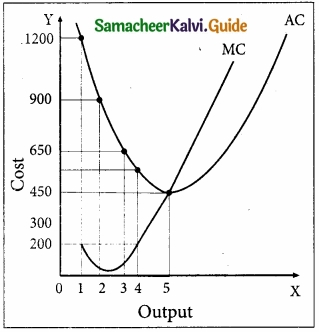1. When AC is falling, MC lies below AC.
2. When AC becomes constant, MC also becomes equal to it.
3. When AC starts increasing, MC lies above the AC.
4. MC curve always cuts AC at its minimum point from below.Question 33.
Write a short note on Marginal Revenue.

1. Marginal Revenue [MR] is the addition to the total revenue by the sale of an additional unit of a commodity.
2. MR can be found out by dividing change in total revenue by the change in quantity sold out.
3. MR = ∆TR/∆Q where MR denotes Marginal Revenue, ∆TR denotes a change in Total Revenue and ∆Q denotes a change in total quantity.
4. The other method of estimating MR is:

MR = TRn – TRn-1, (or) TRn+1 – TRn
Where MR denotes Marginal Revenue,
TRn denotes total revenue of nth item,
TRn-1 denotes Total Revenue of n – 1th item and
TRn+1 denotes Total Revenue of n + 1th item.
If TR = PQ,
MR = dTR/dQ = P, which is equal to AR.Question 34.
Discuss the Long run cost curves with a suitable diagram?

1. In the long run, all factors of production become variable. The existing size of the firm can be increased in the case of the long run. There are neither fixed inputs nor fixed costs in the long run.
2. LAC is given in the diagram.
3. Long-run average cost (LAC) is equal to long-run total costs divided by the level of output.

LAC = LTC/Q where LAC denotes Long-Run Average Cost, LTC denotes Long-run Total Cost and Q denotes the quantity of output.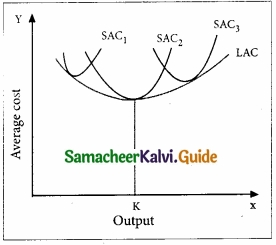The LAC curve is derived from short-run average cost curves. It is the locus of points denoting the least cost curve of producing the corresponding output. The LAC curve is called a ‘Plant Curve’ or ‘Boat shape Curve’ or ‘Planning Curve’ or ‘Envelop Curve’.

Part – D

Question 35.
If total cost = 10 + Q3, find out AC, AVC, TFC, AFC when Q = 5?
TC = 10 + Q3
AC = $$\frac { TC }{ Q }$$
AC = $$\frac{10+Q^{3}}{Q}$$
If Q=5, Q = 5 × 5 × 5 = 125
AC = $$\frac { 10 + 125 }{ 5 }$$ = $$\frac { 135 }{ 5 }$$ = 27

AVC:
TC = 10 + Q3
TC = TFC + TVC
TVC = Q3
AVC = $$\frac { TVC }{ Q }$$
= $$\frac{Q^{3}}{Q} = Q$$2
If Q = 5, then AVC = 52
AVC = 25

TFC:
TC = 10 + Q3
TC = TFC + TVC
TFC = 10

AFC:
AFC = $$\frac { TFC }{ Q }$$
= $$\frac { 10 }{ 5 }$$
AFC = 2Question 36.
Discuss the short-run cost curves with a suitable diagram?
Short-run Cost Curves:
Total Fixed Cost (TFC):
All payments for the fixed factors of production are known as Total Fixed Cost. A hypothetical TFC is shown in the below table and the diagram.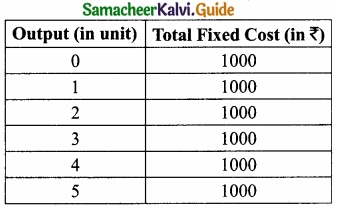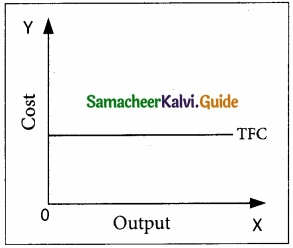For instance if TC = Q3 – 18Q2 + 91Q + 12. the fixed cost here is 12. That means, if Q is zero, the total cost will be 12, hence fixed cost.
It could be observed that TFC does not change with output. Even when the output is zero, the fixed cost is ₹1000. TFC is a horizontal straight line, parallel to X-axis.

Total Variable Cost (TVC):
All payments to the variable factors of production are called Total Variable Cost. Hypothetical TVC is shown in the below table and diagram.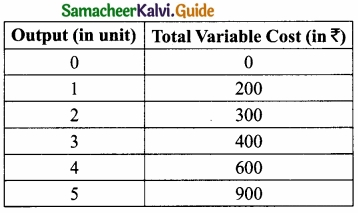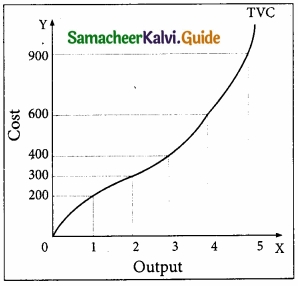In the diagram, the TVC is zero when nothing is produced. As output increases, TVC also increases. TVC curve slopes upward from left to right.

For instance in TC = Q3 – 18 Q2 + 91Q + 12, variable cost, TVC = Q3 – 18Q2 + 91Q

Total Cost Curves:
Total Cost means the sum total of all payments made in the production. It is also called as Total Cost of Production. Total cost is the summation of Total Fixed Cost (TFC) and Total Variable Cost (TVC). It is written symbolically as TC = TFC + TVC.

For example, when the total fixed cost is ₹1000 and the total variable cost is ₹200 then the Total cost is = ₹1200 (₹1000 + ₹200).
If TFC = 12 and
TVC = Q3 – 18Q2 + 91Q
TC = 12 + Q3 – 18Q2 + 91Q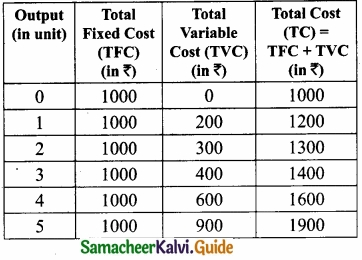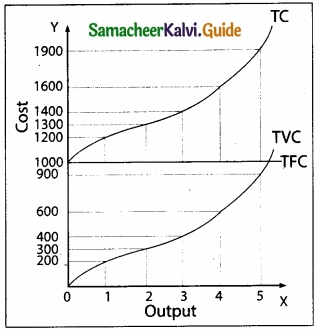Average Fixed Cost (AFC):
Average Fixed Cost refers to the fixed cost per unit of output. It is obtained by dividing the total fixed cost by the quantity of output. AFC = TFC / Q where AFC denotes average fixed cost, TFC denotes total fixed cost and Q denotes the quantity of output. For example, if TFC is 1000 and the quantity of output is 10, the AFC is ₹100, obtained by dividing ₹1000 by 10. TVC is shown in the below table and diagram?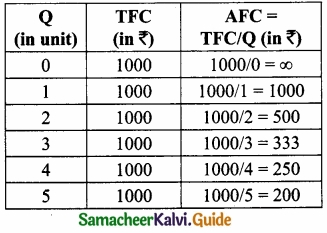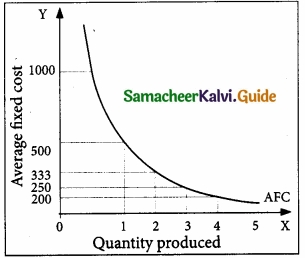It is to be noted that-

1. AFC declines as output increases, as fixed cost remains constant.
2. AFC curve is downward sloping throughout its length, never touching the X and Y-axis. It is asymptotic to both axes.
3. The shape of the AFC curve is a rectangular hyperbola.

Average Variable Cost (AVC):
Average Variable Cost refers to the total variable cost per unit of output. It is obtained by dividing total variable cost (TVC) by the quantity of output (Q). AVC = TVC / Q where, AVC denotes Average Variable cost, TVC denotes total variable cost and Q denotes the quantity of output. For example, When the TVC is ₹300 and the quantity produced is 2, the AVC is ₹150,
(AVC = 300/2 = 150) AVC is shown in the below table and diagram.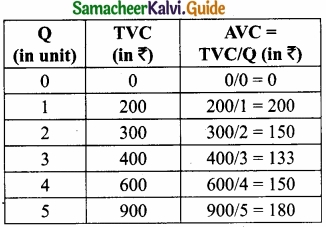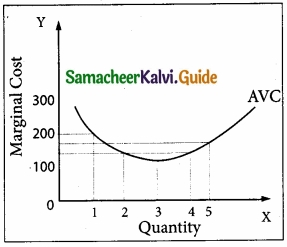Average Total Cost (ATC) or Average Cost (AC):
Average Total Cost refers to the total cost per unit of output.

It can be obtained in two ways.

1. By dividing the firm’s total cost (TC) by the quantity of output (Q). ATC = TC / Q.
For example, if TC is ₹1600 and the quantity of output is Q = 4, the Average Total Cost is ₹400. (ATC = 1600/4 = 400)
If ATC is Q3 – 18Q2 + 91Q + 12, then AC = Q2 – 18Q + 91 + 12/Q

2. By ATC is derived by adding together Average Fixed Cost (AFC) and Average Variable Cost (AVC) at each level of output. ATC = AFC + AVC.
For example, when Q = 2, TFC = 1000, TVC = 300; AFC = 500; AVC = 150; ATC = 650. ATC or AC is shown in the below table and diagram.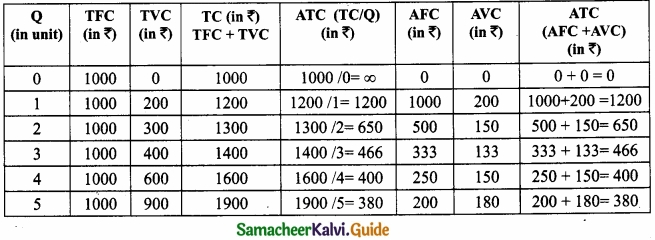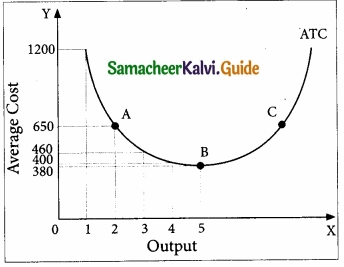Marginal Cost (MC):
Marginal Cost is the cost of the last single unit produced. It is defined as the change in total costs resulting from producing one extra unit of output. In other words, it is the addition made to the total cost by producing one extra unit of output.

Marginal cost is important for deciding whether any additional output can be produced or not. MC = ∆TC/∆Q where MC denotes Marginal Cost, ∆TC denotes a change in total cost and ∆Q denotes a change in total quantity.

For example, a firm produces 4 units of output and the Total cost is ₹1600. When the firm produces one more unit (4 + 1 = 5 units) of output at the total cost of ₹1900, the marginal cost is ₹300.
MC = 1900 – 1600 = ₹300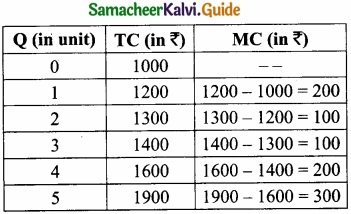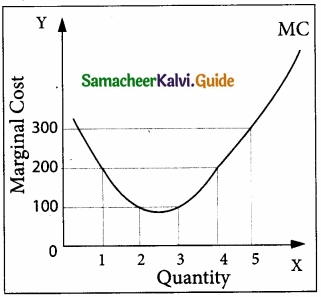Question 37.
Bring out the relationship between AR and MR curves under various price conditions?
Relationship between AR and MR Curves:
If a firm is able to sell additional units at the same price then AR and MR will be constant and equal. If the firm is able to sell additional units only by reducing the price, then both AR and MR will fall and be different.

Constant AR and MR (at Fixed Price):
When price remains constant or fixed, the MR will be also constant and will coincide with AR. Under perfect competition as the price is uniform and fixed, AR is equal to MR and their shape will be a straight line horizontal to X-axis. The AR and MR Schedule under constant price is given in the below table and in the diagram.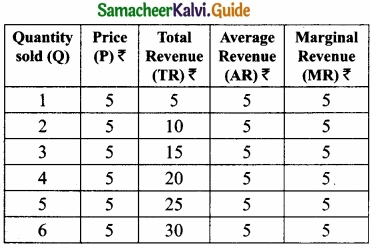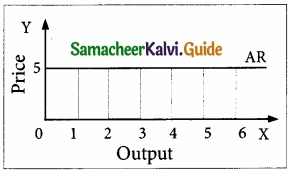Declining AR and MR (at Declining Price):
When a firm sells large quantities at lower prices both AR and MR will fall but the fall in MR will be steeper than the fall in the AR.

It is to be noted that MR will be lower than AR. Both AR and MR will be sloping downwards straight from left to right. The MR curve divides the distance between AR Curve and Y-axis into two equal parts. The decline in AR need not be a straight line or linear. If the prices are declining with the increase in quantity sold, the AR can be non-linear, taking a shape of concave or convex to the origin.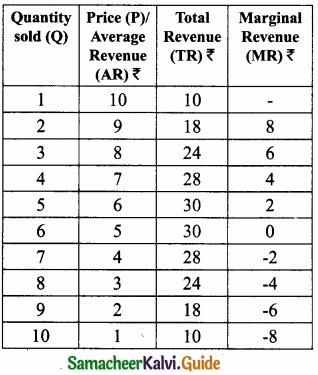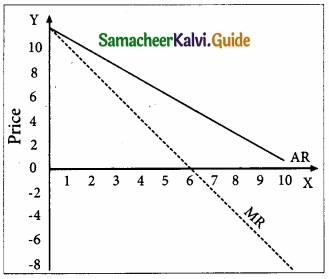### Samacheer Kalvi 11th Economics Cost and Revenue Analysis Additional Important Questions and Answers

Part – A

Multiple Choice Questions:

Question 1.
Real cost is _______
(a) Pain and sacrifice
(b) Subjective
(c) Efforts and sacrifice
(d) All the above
(d) All the aboveQuestion 2.
Social Cost is those costs ………………………
(a) Not borne by the firms
(b) Incurred by the society
(c) Health hazards
(d) All the above
(d) All the above

Question 3.
Economic profit is ______
(a) TR-TC
(b) TC-TR
(c) AC-MC
(d) None
(a) TR-TCQuestion 4.
Profit is the difference between Total Revenue and ………………………
(a) Total Cost
(b) Total Variable
(c) Total Fixed Cost
(d) Total Marginal Cost
(a) Total Cost

Question 5.
How can you calculate the average cost?
(a) TVC + TFC
(b) TC – AC
(c) TC / Q
(d) AC / Q
(c) TC / Q

Question 6.
What is an envelope curve?
(a) Planning curve
(b) Long-run cost curve
(c) U – shape curve
(d) V – shape curve
(a) Planning curveQuestion 7.
Social cost is _______
(a) Not borne by the firm
(b) Borne by the society
(c) Air pollution by the firm
(d) All the above
(d) All the above

Question 8.
Implicit cost is also known as ……………………….
(a) Explicit Cost
(b) Economic Cost
(c) Social Cost
(d) Imputed Cost
(d) Imputed Cost

Question 9.
Long-run average cost curve can also be called as _______
(a) Planning curve
(b) Envelope curve
(c) Boat-shaped curve
(d) All the above
(d) All the aboveQuestion 10.
How will you calculate AR?
(a) AR = $$\frac{TR}{Q}$$
(b) AR = $$\frac{TVR}{Q}$$
(c) AR = $$\frac{TFR}{Q}$$
(d) AR = $$\frac{TAR}{Q}$$
(a) AR = $$\frac{TR}{Q}$$

Part – B

Answer the following questions in one or two sentences.

Question 1.
What does money cost?
Production cost expressed in money terms is called money cost.
(OR)
The total money expenses incurred by a firm in producing a commodity.Question 2.
Define Floating Cost?
Floating cost refers to all expenses that are directly associated with business activities but not with asset creation. It does not include the purchase of raw material as it is part of current assets. It includes payments like wages to workers, transportation charges, fees for power, and administration. Floating cost is necessary to run the day-to-day business of a firm.

Question 3.
What is the social cost?
Social cost refers to the total cost borne by society due to the production of a commodity. (OR) Alfred Marshall defined the term social cost to represent the efforts and sacrifices undergone by the various members of the society in producing a commodity.

Part – C

Answer the following questions in one paragraph.

Question 1.
Bring out the distinction between short-run and long Run?

 Short Run Long Run 1. Period of one year. Period of more than one year. 2. Atleast one of the inputs is fixed. All the inputs are variable. 3. Demand is the main determinant in fixing the price. Supply is the main determinant in fixing the price.Question 2.
How is the average variable cost be calculated?
It refers to the total variable cost per unit of output. It is obtained by dividing the total variable cost by the quantity of output.
AVC = $$\frac { TVC }{ Q }$$
(Eg.) If TFC is 300;
Q = 2 Find AVC
= $$\frac { 300 }{ 2 }$$
AVC = 150.

Question 3.
What is meant by Social Cost for example?

1. Social cost refers to the total cost borne by the society due to the production of a commodity.
2. Alfred Marshall defined the term social cost to represent the efforts and sacrifices undergone by the various members of the society in producing a commodity.
3. Social Cost is the cost that is not borne by the firm but incurred by others in the society.
4. For example, large business firms cause air pollution, water pollution, and other damages ‘ in a particular area that involve a cost to society.
5. These costs are treated as social costs. It is also called External Cost.Question 4.
Write a short note on Average Revenue?
Average revenue is the revenue per unit of the commodity sold. It is calculated by dividing the Total Revenue (TR) by the number of units sold (Q)
AR = TR /Q ; if TR = PQ, AR = PQ/Q = P
AR denotes Average Revenue, TR denotes Total Revenue and Q denotes Quantity of unit sold.
For example, if the Total Revenue from the sale of 5 units is ₹30, the Average Revenue is ₹6. (AR = 30/5 = 6) It is to be noted that AR is equal to Price.
AR = TR/Q
= PQ/Q
= P

Part – D

Question 1.
Write a short note on Total Revenue?
Total revenue is the amount of income received by the firm from the sale of its products. It is obtained by multiplying the price of the commodity by the number of units sold.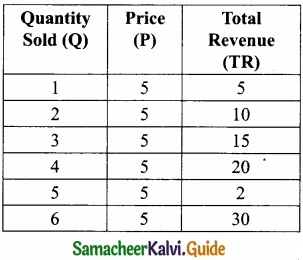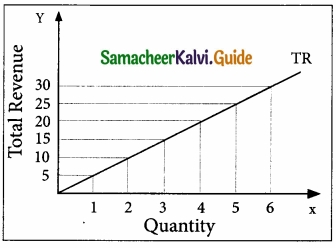TR = P × Q
where TR denotes Total Revenue, P denotes Price, and Q denotes Quantity sold.
For example, a cell – phone company sold 100 cell – phones at the price of ₹500 each.
TR is ₹50,000. (TR = 500 × 100 = 50,000).
When the price is constant, the behaviour of TR is shown in the above table and diagram, assuming P = 5.
When P = 5; TR = PQ
When the price is declining with an increase in quantity sold. (Eg. Imperfect Competition on the goods market) the behaviour of TR is shown in the table and diagram. TR can be obtained from the Demand function: If Q = 11 – P,

When P = 1, Q = 10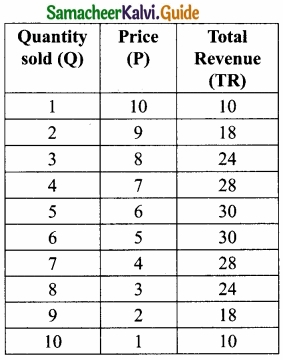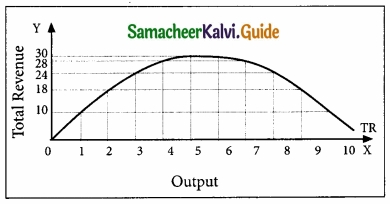TR = PQ = 1 × 10 = 10
When P = 3, Q = 8, TR = 24 When P = 0, Q = 1, TR = 10Question 2.
Bring out the relationship between TR, AR, MR, and Elasticity of demand?
The relationship among TR, AR, and MR Curves:
When marginal revenue is positive, total revenue rises, when MR is zero the total revenue becomes maximum. When marginal revenue becomes negative total revenue starts falling. When AR and MR both are falling, then MR falls at a faster rate than AR.

TR, AR, MR, and Elasticity of demand:
The relationship among AR, MR, and elasticity of demand (e) is stated as follows.
MR = AR (e – 1/e)

The relationship between the AR curve and MR curve depends upon the elasticity of the AR curve [AR = DD = Price]

1. When the price elasticity of demand is greater than one, MR is positive and TR is increasing.
2. When the price elasticity of demand is less than one, MR is negative and TR is decreasing.
3. When price elasticity of demand is equal to one, MR is equal to zero and TR is maximum and constant.

It is to be noted that, the output range of 1 to 5 units, the price elasticity of demand is greater than one according to the total outlay method. Hence, TR is increasing and MR is positive.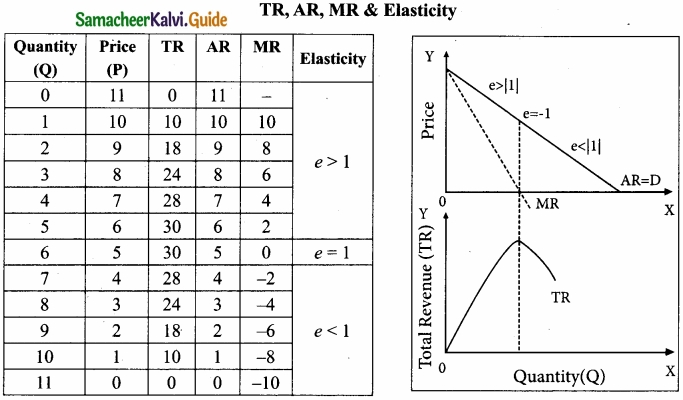1. At the output range of 5 to 6 units, the price elasticity of demand is equal to one. Hence, TR is maximum and MR is equaled to zero.
2. At the output range of 6 units to 10 units, the price elasticity of demand is less than unity. Hence, TR is decreasing and MR is negative.